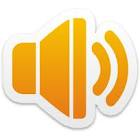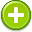#divisible 中文解釋 wordnet sense Collocation Usage
/diˈvizəbəl/,Font size:Capable of being divided,
1. Capable of being divided
• - the marine environment is divisible into a number of areas
2. (of a number) Capable of being divided by another number without a remainder
• - 24 is divisible by 4

1. capable of being or liable to be divided or separated; "even numbers are divisible by two"; "the Americans fought a bloody war to prove that their nation is not divisible"
2. (divisibility) the quality of being divisible; the capacity to be divided into parts or divided among a number of persons
3. In mathematics, a divisor of an integer , also called a factor of , is an integer which divides without leaving a remainder.
4. (Divisibility) the extent to which a new product can be tested in a limited scale purchase.
5. (Divisibility) divisible, part, component, constituent, element, factor, fraction, fragment, ingredient, member, quantum, section, sector, segment, disvision, piece, portion, compartment, region, domain, whole, entire.
6. The smallest unit of space that a landlord would demise from a larger unit and make available to a tenant. Also known as minimum divisible.
7. A number is divisible by another number if it goes into the number evenly with no remainder
8. Capable of being divided, especially with no remainder